Ch 7. Rigid Body Energy Methods Multimedia Engineering Dynamics Rot. Work & Energy Conservation of Energy
 Chapter - Particle - 1. General Motion 2. Force & Accel. 3. Energy 4. Momentum - Rigid Body - 5. General Motion 6. Force & Accel. 7. Energy 8. Momentum 9. 3-D Motion 10. Vibrations Appendix Basic Math Units Basic Equations Sections Search eBooks Dynamics Fluids Math Mechanics Statics Thermodynamics Author(s): Kurt Gramoll ©Kurt GramollDYNAMICS - THEORY

General Work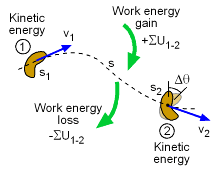Energy Balance

 Force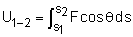Moment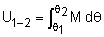Gravity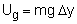Friction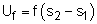Spring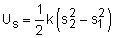Rigid Body Work - Energy Terms

The Principle of Work and Energy equates the total work performed on a body to the change in kinetic energy,

Σ Work = Δ Kinetic Energy

Another way to express this concept is

 T1 + ΣU1-2 = T2

where
T1 = kinetic energy (translational and rotational)
at position 1
T2 = kinetic energy (translational and rotational)
at position 2
ΣU1-2 = total change in energy (i.e. work)
between positions 1 and 2

Work on a rigid body is the same as work on a particle, with the addition of rotational energy. Recall from Particle Energy Methods section, there are various ways to model energy for a particle. These are listed in the table at the left.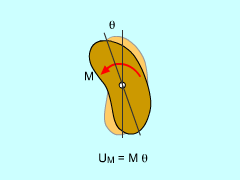Rotating Rigid Body
Work of a Force Couple, or Moment

The work of two parallel, non-collinear forces in opposite directions with equal magnitudes forms a couple and moment. This moment generate energy similar to a linear force through a distance. However, the distance is now the angle of rotation.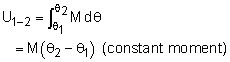Kinetic Energy for a Rotating Body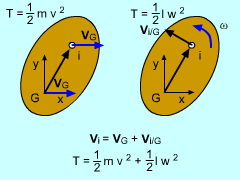Rotational and Translational
Kinetic Energy

For a particle that has no rotation, the kinetic energy is simply,

T = 1/2 m v2

This equation can be applied to every particle that makes up a rigid body,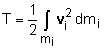However, if the particle is a rigid body with dimensions, then the velocity needs to be written as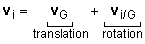where G is the center of gravity. The second terms models the rotation of the object. Substituting vi into the kinetic equation and noting that a magnitude of vi/G = ri/G ω gives

 T = 1/2 m vG2 + 1/2 IG ω2

If the rigid body has a fixed point, then the equation becomes

T = 1/2 Io ω2

where Io is moment of inertia about the fixed point.

Practice Homework and Test problems now available in the 'Eng Dynamics' mobile app
Includes over 400 problems with complete detailed solutions.
Available now at the Google Play Store and Apple App Store.